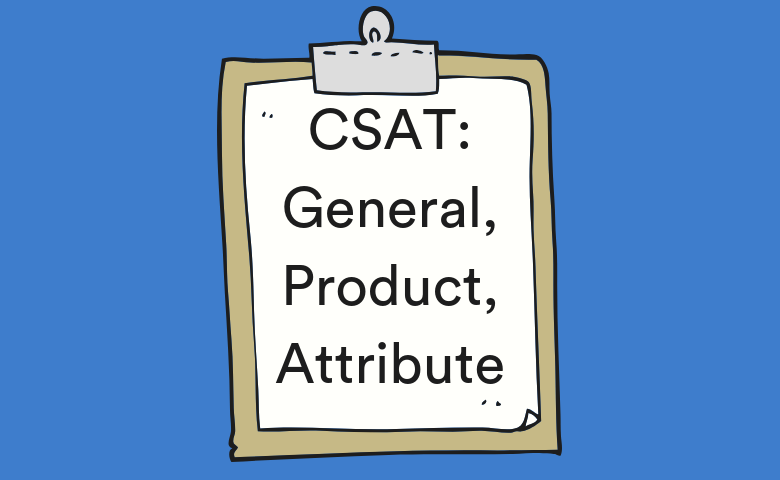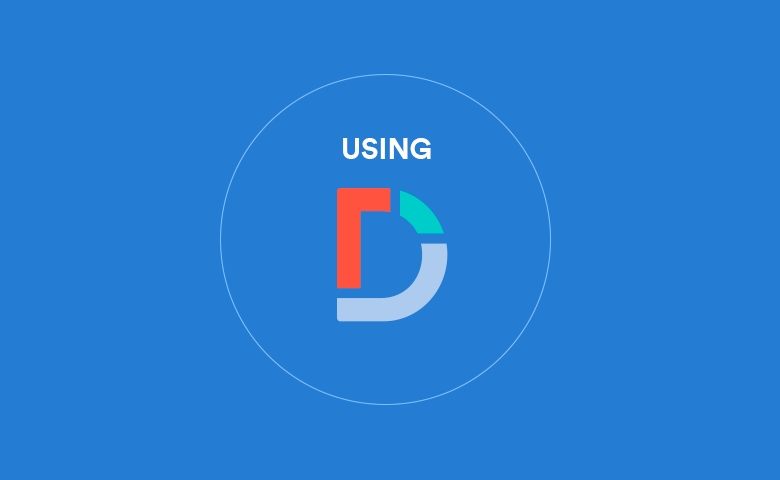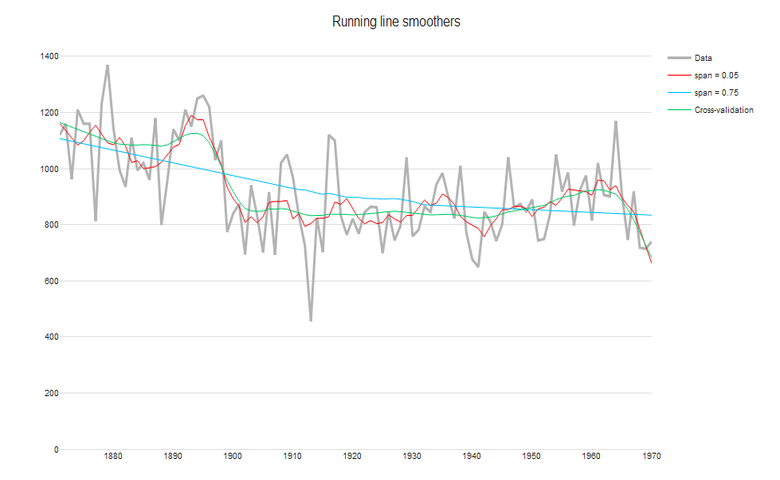# Blog.

## regression.Automatic Removal of Outliers from Regression and GLMs
03 May 2020 | by Tim Bock

A well-known problem with linear regression, binary logit, ordered logit, and other GLMs, is that a small number of rogue observations can cause the results07 February 2020 | by Tim Bock

In this post I describe how to quickly create a quad map in Displayr. The example uses a Shapley Regression to work out the relativeCustomer Satisfaction: General, Product, & Attribute Questions
14 December 2018 | by Madeleine Picard

Rather than just measuring one type of customer satisfaction, it's useful to measure these three aspects of customer satisfaction.How to Interpret Logistic Regression Coefficients
25 October 2018 | by Tim Bock

This post describes how to interpret the coefficients, also known as parameter estimates, from logistic regression (a.k.a. binary logit). Read more.How to do Logistic Regression in Displayr
24 October 2018 | by Tim Bock

This is a practical guide to logistic regression. To get the most out of this post, I recommend you follow along with my instructions andHow to Interpret Logistic Regression Outputs
24 October 2018 | by Tim Bock

Logistic regression (also known as binary logistic regression) is a predictive modeling technique used to predict outcomes involving 2 options. Learn more.What is Logistic Regression?
22 August 2018 | by Justin Yap

Logistic regression is a type of regression analysis used when the dependent variable is binary (i.e., has only two possible outcomes).Smoothing Time Series Data
16 July 2018 | by Carmen Chan

For time-series data, you'll want to separate long-term trends and seasonal changes from random fluctuations. Find out which time smoother to use.What are Variance Inflation Factors (VIFs)?
06 April 2018 | by Tim Bock

The variance inflation factor (VIF) quantifies the extent of correlation between one predictor and the other predictors in a model.Comparing Partial Least Squares to Johnson’s Relative Weights
19 June 2017 | by Tim Bock

Although PLS and Johnson's Relative Weights are both techniques for dealing with correlations between predictors, they give fundamentally different results.The Problem with Using Multiple Linear Regression for Key Driver Analysis: a Case Study of the Cola Market
18 June 2017 | by Tim Bock

Why is Multiple Linear Regression the standard technique taught for Key Driver Analysis when it gets it so wrong? The better method is Johnson’s Relative WeUsing Partial Least Squares to Conduct Relative Importance Analysis in Displayr
16 June 2017 | by Jake Hoare

Partial Least Squares (PLS) is a popular method for relative importance analysis in fields where the data typically includes more predictors than observations. Relative importance analysis5 Ways to Visualize Relative Importance Scores from Key Driver Analysis
26 April 2017 | by Tim Bock

5 ways of presenting the results of key driver analysis techniques, such as Shapley Value, Kruskal Analysis, and Relative Weights.